# Factoring Worksheets

#### What is Factoring?

You can make mathematical expression like multiplicative problem with the help of factoring process. We can also reverse the multiplication method with the help of factoring. You need two numbers for multiplication then, you will have another number. For example, 4 multiply be 8 is equal to 32 as you know multiplicative table. You can also solve all prime factorization methods like 81 = 3 × 3 × 3 × 3. Factoring process also make the factoring out method of greatest common factor. For example, 2× + 10 = 2(x + 5) and 2 is the greatest common factor. You can also strive to solve complicative factor expression such as x2 – 14x – 32, 15x2, - 26x + 11, or 150x3 + 350x2 + 180x + 420. You can also factor algebraic and numeric expressions by using a few specific methods. Factorizing simplest expressions means we are solving numerical expressions. You will have to work hard if you are factorizing 240 number (bigger value) for prime factorization method. You can see the factorization of 240 and other big numbers. You can also solve the factor of algebraic expressions such as x2 + 5x + 6. Factorize more difficult mathematical or algebraic expressions with the help of multiple methods.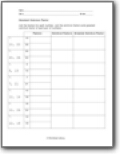###### Greatest Common Factor

List the factors for each number. List the common factors and greatest common factor of each pair of numbers.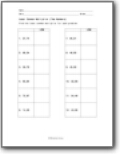###### Least Common Multiplier (Two Numbers)

Find the least common multiplier for each problem.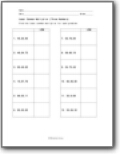###### Least Common Multiplier (Three Numbers)

Find the least common multiplier for each problem.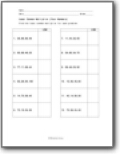###### Least Common Multiplier (Four Numbers)

Find the least common multiplier for each problem.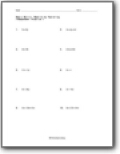###### Rewrite by Factoring

You are given an algebraic statement and you will need to rewrite it even better.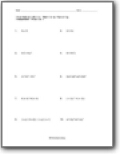###### Rewriting Intermediate Skills

We work with more complex expressions.